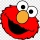Home Communities
IT Knowledge
Inspiration
Languages
EN

# JavaScript - how to use Monte Carlo method to calculate pi constant number?

6 points
Created by:Kia-H
396

Using JavaScript it is possible to calculate surface area (definite integral) in the following way.

## 1. Circle surface area calculation example

Main idea of this method is to:

1. random big amount of points
2. check all time if every next one point is inside a circle or not,
3. compute coefficient by dividing number of points inside of circle by outside one point
4. multiply the coefficient by square surface area - this way from proportion it is possible to get approximated circle surface area,
5. use transformed circle surface area formula to calculate `PI` number.

Note: the longer the simulation is performed, the results are more precised.

``````// ONLINE-RUNNER:browser;

<!doctype html>
<html>
<style>

#my-canvas { border: 1px solid gray; }

</style>
<script>

function randomizePoint(range) {
return range * (Math.random() - 0.5);
}

// This method checks the point is inside circle
// with center at (0, 0) point.
//
var pointRadius = Math.sqrt(pointX * pointX + pointY * pointY);

return true;
}

return false;
}

window.Drawer = function(canvas) {
var context = canvas.getContext('2d');

var xCenter = canvas.width / 2;
var yCenter = canvas.height / 2;

this.clearCanvas = function() {
context.clearRect(0, 0, canvas.width, canvas.height);
};

this.drawPoint = function(x, y, color) {

var tmpX = Math.round(xCenter + x - 1);
var tmpY = Math.round(yCenter + y - 1);

context.strokeStyle = '#fff';
context.fillStyle = color || '#000';

context.fillRect(tmpX, tmpY, 2, 2);
};

this.drawCircle = function(x, y, radius) {

var tmpX = Math.round(xCenter + x - 1);
var tmpY = Math.round(yCenter + y - 1);

context.lineWidth = 0.5;
context.strokeStyle = '#ff0000';
context.fillStyle = '#fff';

context.beginPath();
context.arc(tmpX, tmpY, radius, 0, 2 * Math.PI);
context.stroke();
};
};

</script>
<body>
<canvas id="my-canvas" width="300" height="300"></canvas>
<script>

var canvas = document.querySelector('#my-canvas');

var drawer = new Drawer(canvas);

var squareArea = canvas.width * canvas.height;

var squarePointsCount = 0;
var circlePointsCount = 0;

drawer.clearCanvas();

function makeIteration() {

for(var i = 0; i < 100; ++i) {

// random coordinates from range -150 to +150
var pointX = randomizePoint(canvas.width);
var pointY = randomizePoint(canvas.height);

drawer.drawPoint(pointX, pointY, 'red');

circlePointsCount += 1;
} else {
drawer.drawPoint(pointX, pointY, 'silver');
}

squarePointsCount += 1;
}

// next iterations gives computation better precision
// according to number of randomized points

var randomPointsProportion = circlePointsCount / squarePointsCount;

var computatedCircleArea = randomPointsProportion * squareArea;

var computationError = Math.abs(Math.PI - computatedPI);

console.clear();

console.log('PI number:');
console.log(' Expected: ' + Math.PI);
console.log(' Computed: ' + computatedPI);
console.log(' Error: ' + computationError);
}

setInterval(makeIteration, 100);

</script>
</body>
</html>``````

## References

1. Monte Carlo method - Wikipedia